# Math in Focus Grade 5 Cumulative Review Chapters 5 to 7 Answer Key

Practice the problems of Math in Focus Grade 5 Workbook Answer Key Cumulative Review Chapters 5 to 7 to score better marks in the exam.

## Math in Focus Grade 5 Cumulative Review Chapters 5 to 7 Answer Key

Concepts and Skills

Write the next three terms of each pattern. Then tell the rule you used. (Lesson 5.1)

Question 1.
1, 5, 25, 125, ____, ___, ____,…
Rule: __________________
1, 5, 25, 125, 625, 3125, 15625.
Rule: Multiply each number by 5 to get the next three patterns.

Question 2.
8, 14, 20, 26, ___, ___, ____,…
Rule: __________________
8, 14, 20, 26, 32, 38, 44.
Rule: Add 6 to each number to get the next three patterns.

Question 3.
6,236, 6,030, 5,824, 5,618. ___, ___, ___, …
Rule: ________________________
6236, 6030, 5824, 5618, 5412, 5206, 5000.
Rule: Subtract 206 from each number to get the next three patterns.

Complete the table. (Lesson 5.1)

Question 4.
The width of a rectangle is 7 centimeters long. Complete the table to find the area as the length increases.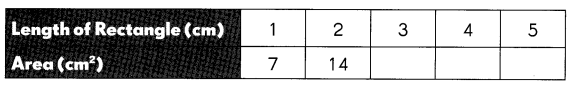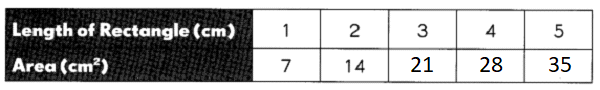Explanation:
Given,
The width of a rectangle is 7 centimeters long,
Multiply the length of the rectangle with 7 to get the area as the length increases.

Complete the table and answer the questions that follow. (Lesson 5.1)

Question 5.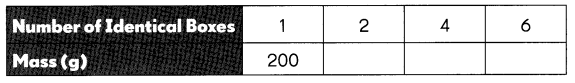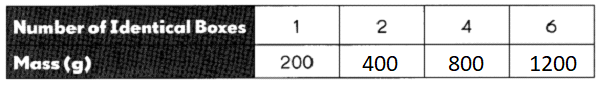a. How much do 5 boxes weigh? ____
5 boxes weigh 1000.
Explanation:
Given,
Each box weighs 200,
Multiply 5 boxes with 200 then we get 1000.

b. How many boxes weigh 600 grams? _____
Explanation:
Given,
Each box weighs 200,
Multiply 6 boxes with 200 then we get 1200.

c. If 100 grams cost $5, how much will 5 boxes cost? __________ Answer:5 boxes cost$5000.
Explanation:
Given,
Each 100 grams cost $5, 5 boxes contain 1000 grams, To get the cost of 5 boxes 1000 grams should be multiplied by 5 then we get 5000 as the total sum, The cost of 5 boxes is$5000.

Evaluate each expression for z = 5. (Lesson 5.2)

Question 6.
x + 9

Explanation:
Given,
= x + 9, if x = 5
= 5 + 9
= 14

Question 7.
16 – x

Explanation:
Given,
= 16 – x, if x = 5
= 16 – 5
= 11

Question 8.
4x

Explanation:
Given,
= 4x, if x = 5
= 4(5)
= 20

Question 9.
$$\frac{x}{5}$$

Explanation:
Given,
=> x / 5, if x = 5
=> 5 / 5
=> 1

Simplify each expression. (Lesson 5.3)

Question 10.
y + 3y

Explanation:
Given,
=> y + 3y
=> 4y

Question 11.
a + a – 2

Question 12.
3b + 5b – 2b
Given,
=> 3b + 5b – 2b
=> 8b – 2b
=> 6b

Question 13.
8c + 6 – 1 – c
Given,
=> 8c + 6 – 1 – c
=> 8c – c + 6 – 1
=> 7c + 6 – 1
=> 7c + 5

Complete with =, >, or < for d = 7. (Lesson 5.4)

Question 14.
d + 7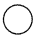15
Given,
=> 7 + 7 = 15
=> 14 < 15

Question 15.
3d – 1011
Given,
=> 3d – 10 = 11
=> 3(7) – 10 = 11
=> 21 – 10 = 11
=> 11 = 11

Question 16.
2d + 63d – 2
Given,
=> 2d + 6 = 3d – 2
=> 2(7) + 6 = 3(7) – 2
=> 14 + 6 = 21 – 2
=> 20 > 19

Question 17.
(35 ÷ d) + 5d
Given,
35 / d, where d = 7,
=> 35 / 7 + 5 = 7,
=> 5 + 5 = 7,
=> 10 > 7

Solve each equation. (Lesson 5.4)

Question 18.
2e = 8
e = ________
Given,
=> 2e = 8
=> e = 8/2
=> e = 4

Question 19.
3f + 3 = 18
f = _________
Given,
=> 3f + 3 = 18,
=> 3f = 18 – 3
=> 3f = 15
=> f = 15/3
=> f = 5

Question 20.
6g – 5 = 2g + 3
g = _____
6g – 5 = 2g + 3
=> 6g – 2g = 3 + 5
=> 4g = 8
=> g = 8 / 4
=> g = 2.

Question 21.
4h – 11 = h + 16
h = ____
Given,
=> 4h – 11 = h + 16
=> 4h – h = 16 + 11
=> 3h = 27
=> h = 27 / 3
=> h = 9

Find the area of each rectangle. (Lesson 6.1)

Question 22.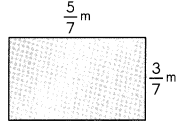Area: _____
Given,
=> Length = 5 / 7 m, Width = 3 / 7 m,
=> Area of rectangle = Length x Width
=> A = 5 / 7 x 3 / 7
=> A = 15 / 49

Question 23.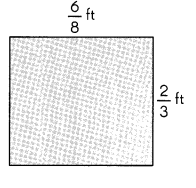Given,
=> Length = 6 / 8 ft, Width = 2 / 3 ft,
=> Area of rectangle = Length x Width
=> A = 6 / 8 x 2 / 3
=> A = 12 / 24
=> A = 1 / 2

Question 24.
A rectangular tray was placed on a square mat. The tray measures $$\frac{3}{4}$$ feet by $$\frac{5}{6}$$ feet. what is the area of the tray.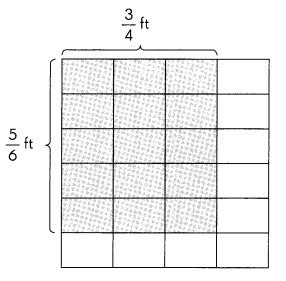Answer: Area of the rectangular tray = 5 / 8

Explanation:
Given,
=> Area of rectangular tray = Length x Width
=> A = 3 / 4 x 5 / 6
=> A = 15 / 24
=> A = 5 / 8

Complete to give both the base and the height in each triangle. (Lesson 6.2)

Question 25.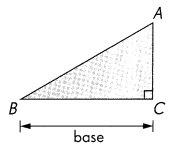Base: ____
Height: _____
Answer: Base = BC, Height = AC

Question 26.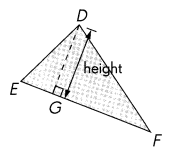Height: _____
Base: ____
Answer: Base = EF, Height = DG

Question 27.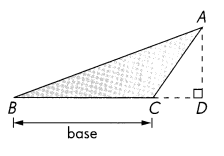Base: ____
Height: _____

Question 28.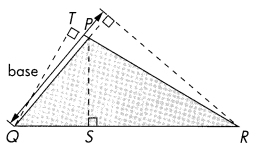Base: ____
Height: _____
Answer: Base = PQ, Height = PS

Find the area of each shaded triangle. (Lesson 6.3)

Question 29.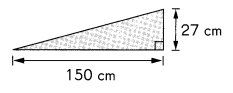Area = ___
Answer: Area of the triangle = 2025

Explanation:
Given,
Area of triangle = 1/2 x base x height
=> 1/2 x 150 x 27
=> 1 x 75 x 27
=> 2025

Question 30.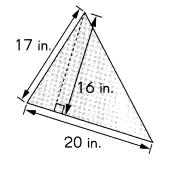Area = ___
Answer: Area of triangle = 160 square inches

Explanation:
Given,
=> Area of triangle = 1/2 x base x height
=> A = 1/2 x 20 x 16
=> A = 1 x 10 x 16
=> A = 160 square inches

Find the area of each shaded triangle. (Lesson 6.3)

Question 31.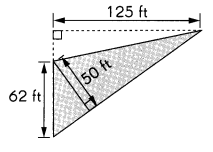Area = ____
Answer: Area of triangle = 3875 square ft

Explanation:
Given,
Area of triangle = 1/2 x base x height
=> A = 1/2 x 125 x 62
=> A = 1 x 125 x 31
=> A = 3875 square ft

Question 32.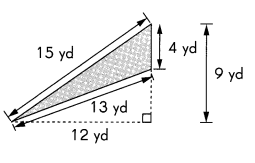Area = ____
Answer: Area of triangle = 26 square yards

Explanation:
Given,
=> Area of triangle = 1/2 x base x height
=> A = 1/2 x 13 x 4
=> A = 1 x 13 x 2
=> A = 26 sqaure yards.

Question 33.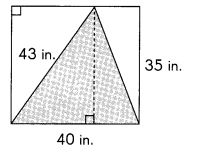Area = ____
Answer: Area of triangle = 700 square inches

Explanation:
Given,
=> Area of triangle = 1/2 x base x height
=> A = 1/2 x 40 x 35
=> A = 20 x 35
=> A = 700 square inches

Question 34.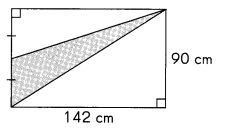Explanation:
Given,
=> Area of triangle = 1/2 x base x height
=> A = 1/2 x 142 x 90
=> A = 1 x 71 x 90
=> A = 6390 square cm

Find the total area of the shaded parts. (Lesson 6.3)

Question 35.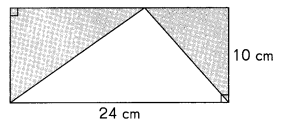Area = ____
Answer: Area of shaded parts in the triangle = 120 square cm

Explanation:
Given,
Area of triangle = 1 / 2 x base x height
=> A = 1 / 2 x 24 x 10
=> A = 1 x 24 x 5
=> A = 120 square cm

Complete. (Lesson 7.1)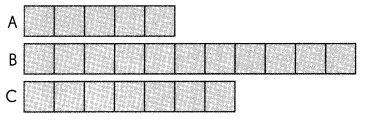Question 36.
The ratio of the length of A to the length of B is _________ : ______
Explanation:
Given,
Length of A is 5,
Length of B is 11,
Ratio of the length of A to the length of B is 5 : 11.

Question 37.
The ratio of the length of C to the length of A is _________ : _____
Explanation:
Given,
Length of C is 7,
Length of A is 5,
Ratio of the length of C to the length of A is 7 : 5

Question 38.
The ratio of the length of B to the total length of A, B, and C is __ : ____
Explanation:
Given,
Length of B is 11,
Total length of A, B and C is by adding 5 with 11 we get 16 and add 16 to 7 we get 23,
Ratio of the length of B to th total length of A, B and C is 11 : 23.

Complete. (Lesson 7.2)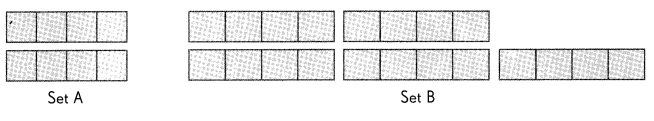Question 39.
The ratio of the number of squares in Set A to the number of squares in Set B is ______ : ___.
Explanation:
Given,
Number of squares in Set A is 8,
Number of squares in Set B is 20,
Ratio of the number of squares in Set A to the number of squares in Set B is 8 : 20

Question 40.
The ratio of the number 0f groups in Set A to the number of groups in Set B is ______ : ____.
Explanation:
Number of groups in Set A is 2,
Number of groups in Set B is 5,
Ratio of the number of groups in Set A to the number of groups in Set B is 2 : 5

Question 41.
___________ : ___________ ___________ : ___________ in simplest form.
Answer: 8 : 20 = 4 : 10 = 2 : 5
Explanation:
Numbers 8 and 20 are divisible by 2 we get 4 : 10,
4 and 10 are again divisible by 2 we get 2 : 5,
To get into simplest form 8 and 20 should be divisible by 2.

Find the missing number or term in each set of equivalent ratios. (Lesson 7.2)

Question 42.
7 : 4 = 21: ______
Answer: 7 : 4 = 21 : 12
Explanation:
Given,
Both the numbers 7 and 4 can be multiplied by 3, we get 21 : 12

Question 43.
5 : 9 = ______ : 63
Answer: 5 : 9 = 35 : 63
Explanation:
Given,
Both the numbers 5 and 9 can be multiplied by 7, we get 35 : 63

Question 44.
18 : 21 = 6: _____
Answer:18 : 21 = 6 : 7
Explanation:
Given,
Both the numbers 18 and 21 can be divided by 3, we get 6 : 7.

Question 45.
108 : 72 = _____ : 6
Answer: 108 : 72 = 9 : 6
Explanation:
Given,
Both the numbers 108 and 72 can be divided by 12, we get 9 : 6.

Complete. (Lesson 7.4)

The heights of two buildings are shown.

Question 46.
The ratio of the height of Math Plaza to the height of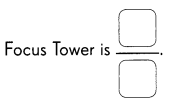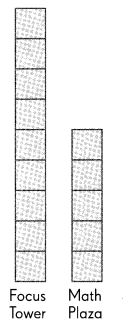Question 47.
The height of Math Plaza is ___ times the height of Focus Tower.
Answer: The height of Math plaza is 5 / 9 times the height of focus tower

Explanation:
Given,
Focus tower height as 9,
Math Plaza height as 5,
Therefore, the height of Math plaza is 5 / 9 times the height of focus tower.

Question 48.
The height of Focus Tower is ___ times the height of both buildings.
Answer: The height of focus tower is 9 / 14 times the height of both buildings

Explanation:
Given,
Height of Focus tower is 9,
Height of Math plaza is 5,
By adding 9 with 5 we get 14,
Therefore, the height of focus tower is 9 / 14 times the height of both buildings.

Express each ratio in simplest form. (Lesson 7.5)

Question 49.
8 : 12 : 24 = ____ : ____ : ____
Answer: 8 : 12 : 24 = 2 : 3 : 4
Explanation:
Given,
Numbers 8, 12 and 24 are divisible by 4 to get into simplest form as 2 : 3 : 4.

Question 50.
21 : 9 : 36 = __ : __ : ___
Answer: 21 : 9 : 36 = 7 : 3 : 12
Explanation:
Given,
Numbers 21, 9 and 36 are divisible by 3 tp get into the simplest form as 7 : 3 : 12.

Find the missing numbers or terms in each set of equivalent ratios. (Lesson 7.5)

Question 51.
4 : 6 : 9 = 24: __ : ___
Answer: 4 : 6 : 9 = 24 : 36 : 54
Explanation:
Given,
Ratio 4 : 6 : 9 is multiplied by 6 then we get 24 : 36 : 54

Question 52.
48 : 56 : 28 = ___ : ___ : 7
Answer: 48 : 56 : 28 = 12 : 14 : 7
Explanation:
Given,
Ratio 48 : 56 : 28 is multiplied by 4 then we get 12 : 14 : 7

Problem Solving

Question 53.
Mandy scores b points in a basketball game. Jay scores 3 points less than Mandy. Kareem scores 2 times as many points as Mandy.

a. Find the number of points that Jay scores in terms of b.
Answer: The number of points that Jay scores is b – 3

Explanation:
Given,
Mandy scores b points in a basketball game,
Jay scores 3 points less than Mandy,
Therefore, Jay scores b – 3 points less than Mandy in terms of b.

b. Find the total number of points the three players score in terms of b.
Answer: The total number of points of three players are Mandy’s scores b, Jay scores b – 3, Kareem scores 2b.

Explanation:
Given,
Mandy scores b points, Mandy scores = b points
Jay scores 3 points less than Mandy, Jay scores = b – 3 points,
Kareem scores 2 times as many points as Mandy, Kareem scores = 2b points.

Question 54.
David reads a book that has (3x + 6) pages. Ellen reads a book that has (4x – 4) pages.

a. If x = 7, whose book has more pages?
Answer: David reads a book which has 27 pages and Ellen reads a book which has 24 pages, So David’s book has more pages than Ellen’s book.

Explanation:
Given,
David’s book:                   Ellen’s book:
=> (3x + 6), If x = 7         => (4x – 4), if x = 7
=> (3(7) + 6)                     => (4(7) – 4)
=> 21 + 6                            => 28 – 4
=> 27                                  => 24

b. For what value of x will the two books have the same number of pages?
Answer: If x = 10, then two books have the same number of pages
Explanation:
Given,
David’s book:                     Ellen’s book:
=> (3x + 6), If x = 10        => (4x – 4), if x = 10
=> (3(10) + 6)                    => (4(10) – 4)
=> 30 + 6                            => 40 – 4
=> 36                                   => 36

Question 55.
In the figure, BC = 18 cm and AD = BD. The length of BD is twice the length of BC. Find the area of the shaded triangle ABC.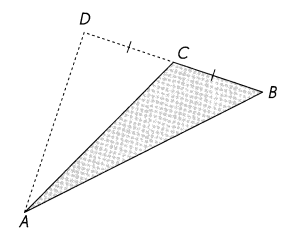Answer: Area of triangle = 324 square cm

Explanation:
Given,
BC = 18 cm,
BD = twice the length of BC,
BD = 2(18) = 36 cm
=> Area of triangle = 1/2 x base x height
=> A = 1/2 x BC x AC
=> A = 1/2 x 18 x 36
=> A = 1 x 9 x 36
=> A = 324 square cm

Question 56.
ABCD is a rectangle with a width of 1 2 centimeters. Its length is twice as long as its width. AE = 12 centimeters and AF = BF. Find the area of the shaded triangle CEF.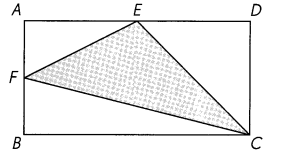Answer: Area of triangle = 144 square cm

Explanation:
Given,
Width = 12 cm,
Length is twice as long as width, L = 2 x 12 = 24 cm,
AE = 12 cm,
AF = BF,
=> Area of triangle = 1/2 x base x height
=> A = 1/2 x 24 x 12
=> A = 1 x 12 x 12
=> A = 144 square cm.

Question 57.
There were 45 pennies in Container A and 79 pennies in Container B at first. Suki took 7 pennies from Container A. She then put them into Container B.

a. What is the ratio of the number of pennies in Container A to that in Container B at first?
Explanation:
Given,
There were 45 pennies in Container A,
Pennies in Container B is 79,
The ratio of the number of pennies in Container A to that in Container B at first is 45 : 79

b. Find the ratio of the number of pennies in Container A to that in Container B in the end. Express your answer in simplest form.
Explanation:
Given,
There were 45 pennies in Container A at first and Suki took 7 pennies from Container A,
By subtracting 7 from 45 we get 38,
Pennies in Container B is 79,
The ratio of the number of pennies in Container A to that in Container B at the end is 38 : 79.

Question 58.
Peggy cycles 3 times as far as Dakota.
a. Find the ratio of the distance that Peggy cycles to the distance that Dakota cycles. Give your answer in fraction form.
Answer: 3 : 1 or 3 / 1 is the ratio of the distance that Peggy cycles to the distance that Dakota cycles.
Explanation:
Given,
Peggy cycles 3 times as far as Dakota,
So Peggy cycles are considered as 3,
Dakota cycle is considered as 1,
Therefore, the ratio of the distance that Peggy cycles to the distance that Dakota cycles is 3 : 1.

b. How many times the combined distance is the distance that Peggy cycles?
Answer: Peggy goes 3 parts of the total distance compared to 1 part for Dakota,
so Peggy accounts for 3/4 of the entire distance,
Dakota does the other part is 1/4.

Question 59.
A company makes yearly donations to Charities A, B, and C in the ratio 3 : 7 : 9. It donates $5,096 to Charity B in a year. a. How much does it donate to Charity A in a year? Answer: Company donates$2,184 to Charity A in a year.
Explanation:
Given,
A company makes yearly donations to Charities A, B and C in the ratio 3 : 7 : 9,
It donates $5,096 to Charity B in a year, The ratio of A / B is 3 / 7 A / B = 3 / 7 = A / 5,096 Need to cross multiply, then we get 7 A = 3 x 5,096 7 A = 15,288 A = 15288 / 7 A = 2,184 b. How much does it donate to all three charities in a year? Answer: Company donates to all three charities in a year is$13,832.
Explanation:
Given,
Company donates donation to Charity A is 2184,
Company donates donation to Charity B is 5096,
Company donates donation to Charity C is 6552,
By adding 2184, 5096 and 6552 then we get 13,832.

Question 60.
The ratio of the number of boys to the number of girls in a camp is 3 : 7. There are 24 boys in the camp.

a. How many girls are there in the camp?
Answer: 56 number of girls are there in the camp.
Explanation:
Given,
The ratio of the number of boys to the number of girls in a camp is 3 : 7,
There are 24 boys in the camp,
By multiplying 3 and 7 with 8 we get 24 : 56,
Therefore, there are 24 boys and 56 girls in the camp.

b. The camp fee is $50 per person. Find the total amount of fees the girls pay. Answer: The total amount of fees the girls pay is$2800.
Explanation:
Given,
The number of girls in the camp are 56,
The camp fee is $50 per person, By multiplying 56 with 50 we get 2800, Therefore, the total amount of fees the girls pay is$2800.